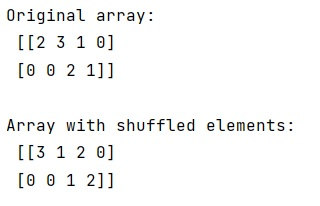# Shuffle non-zero elements of each row in a NumPy array

Learn, how to shuffle non-zero elements of each row in a NumPy array in Python? By Pranit Sharma Last updated : March 31, 2023

Suppose that we are given a numpy array and we need to go through each row of this array and shuffle only the non-zero elements.

## NumPy Array - Shuffling non-zero elements of each row

To shuffle non-zero elements of each row in a NumPy array, we can use the non-in-place numpy.random.permutation() with explicit non-zero indexing. We will simply loop over the array and first extract the index of each nonzero element with the help of which we will index the two-dimensional array so that we can sign it with a numpy random permutation.

Let us understand with the help of an example,

## Python code to shuffle non-zero elements of each row in a NumPy array

```# Import numpy
import numpy as np

# Creating a numpy array
arr = np.array([[2,3,1,0], [0,0,2,1]])

# Display Original array
print("Original array:\n",arr,"\n")

# Shuffling non zero elements
for i in range(len(arr)):
idx = np.nonzero(arr[i])
arr[i][idx] = np.random.permutation(arr[i][idx])

# Display result
print("Array with shuffled elements:\n",arr,"\n")
```

### Output